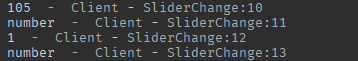# Why does this function need UDim?

Since when did decimal functions need a UDim?! Can anyone explain why this error message is happening

Lines in question:

``````function roundDeci(n: number, decimal)
return math.round(n * 10 ^ decimal) / (10 ^ decimal)
end

label.Text = roundDeci(max*((Dragger.Position.X - Frame.AbsolutePosition.X)/Frame.AbsoluteSize.X), script.Round.Value)
``````

Error message:

script.Round.Value is an integer that gives a parameter

Edit: I tried wrapping script.Round.Value in a UDim.new but it still didn’t work

The function is working as expected; it returns a number. If you can share the rest of your code, we can identify the problem. It seems that you are trying to pass a number instead of a UDim2 value to some UI element.

``````local Player = game:GetService("Players").LocalPlayer

local mouse = Player:GetMouse()
local setting = script.Parent.Name

local IsDragging = false

function roundDeci(n, decimal)
return math.round(n * 10 ^ decimal) / (10 ^ decimal)
end

local Dragger = script.Parent.Slider.Frame:WaitForChild("SliderIndicator")
local beam = script.Parent.Slider.Frame:WaitForChild("SliderBeam")

local parameters = script:WaitForChild("Parameters")
local min = parameters.Value.X
local default = parameters.Value.Y
local max = parameters.Value.Z
local Frame = Dragger.Parent
local label = Frame:WaitForChild("TextLabel")
local defaultpercentage = roundDeci(default/max, script:WaitForChild("Round").Value)
Dragger.Position = UDim2.fromScale(defaultpercentage, Dragger.Position.Y.Scale)
label.Text = roundDeci(max*defaultpercentage, script.Round.Value)

Dragger.MouseButton1Down:Connect(function()
IsDragging = true
end)

Dragger.MouseButton1Up:Connect(function()
IsDragging = false
Player.Settings[setting].Value = roundDeci(max*((Dragger.Position.X - Frame.AbsolutePosition.X)/Frame.AbsoluteSize.X), UDim.new(script.Round.Value))
label.Text = roundDeci(max*((Dragger.Position.X - Frame.AbsolutePosition.X)/Frame.AbsoluteSize.X), UDim.new(script.Round.Value))
end)

mouse.Move:Connect(function()
if IsDragging then
local newPositionX = math.clamp((mouse.X - Frame.AbsolutePosition.X)/Frame.AbsoluteSize.X, 0, 1)
Dragger.Position = UDim2.fromScale(newPositionX, Dragger.Position.Y.Scale)
print(max*((Dragger.Position.X - Frame.AbsolutePosition.X)/Frame.AbsoluteSize.X))
label.Text = roundDeci(max*((Dragger.Position.X - Frame.AbsolutePosition.X)/Frame.AbsoluteSize.X), script.Round.Value)
end
end)

``````

For context, this is a ui slider for a game settings page I am making.

I couldn’t find any related element in this script that would cause the error message you mentioned. In the error message you post, there is a element called “SliderChange” but I couldn’t find it in the script. Could it be that this error is not related to this script?

SliderChange is the name of the script, ignore that

No, there are no connections to other scripts in this script

Oh my bad I’m too tired lol.

Can you post line 39?

``````Dragger.Position = UDim2.fromScale(newPositionX, Dragger.Position.Y.Scale)
``````

If this is the line 39 then I have no idea why it’s happens.

To help you out I managed to narrow it down to this part of line 39:

``````max*(Dragger.Position.X - Frame.AbsolutePosition.X)/Frame.AbsoluteSize.X
``````

When I attempted to print just that, it gave the same error

Yes, that is line 39

Edit: Sorry I was wrong, this is line 39:

``````label.Text = roundDeci(max*((Dragger.Position.X - Frame.AbsolutePosition.X)/Frame.AbsoluteSize.X), script.Round.Value)
``````

Yeah I think I found the problem. You are trying to access X value of UDim2 but you are not telling which X value. You can access the X value like this:

``````Dragger.Position.X.Scale
Dragger.Position.X.Offset
``````

I tried that and did this:

``````label.Text = roundDeci(max*((Dragger.Position.X.Scale - Frame.AbsolutePosition.X.Scale)/Frame.AbsoluteSize.X.Scale), script.Round.Value)
``````

Unfortunately it gave me a new error:

Oh you shouldn’t use scale on “AbsolutePosition”. It’s not a UDim2. You can leave it like before:
`Frame.AbsolutePosition.X`

Ps: Both “AbsolutePosition” and “AbsoluteSize” are Vector2. So you shouldn’t use Scale for them

Ok I did that but it went back to the original error

Can you add these print statements and paste the output here?

``````function roundDeci(n, decimal)
print(n)
print(typeof(n))
print(decimal)
print(typeof(decimal))
return math.round(n * 10 ^ decimal) / (10 ^ decimal)
end
``````Can you share the error again? Maybe line is different?

`Dragger.Position.X` is a UDim value. The position property of UIs are all `UDim2`s, and the X and Y components are `UDim`s.
`Frame.AbsolutePosition.X` is a number value. AbsolutePosition is always measured in `Vector2`, so the X and Y components are `number`s.

That’s your problem. You are trying to do math with two completely unrelated datatypes.

I finally figured out the problem with the script and changed a few things about it other than line 39. I will share the final script in case a person stumbles upon this topic:

Working ui slider system:

``````local Player = game:GetService("Players").LocalPlayer

local mouse = Player:GetMouse()
local setting = script.Parent.Name
local module = require(game.ReplicatedStorage.Modules:WaitForChild("ServerInventory"))
local sound = game.SoundService:WaitForChild("Click2Effect")

local IsDragging = false

function roundDeci(n, decimal)
return math.round(n * 10 ^ decimal) / (10 ^ decimal)
end

local Dragger = script.Parent.Slider.Frame:WaitForChild("SliderIndicator")
local beam = script.Parent.Slider.Frame:WaitForChild("SliderBeam")

local parameters = script:WaitForChild("Parameters")
local min = parameters.Value.X
local default = parameters.Value.Y
local max = parameters.Value.Z
local Frame = Dragger.Parent
local interaction = Frame:WaitForChild("Interaction")
local label = Frame.Parent:WaitForChild("CurrentAmount")
local defaultpercentage = roundDeci(default/max, script:WaitForChild("Round").Value)
Dragger.Position = UDim2.fromScale(defaultpercentage, Dragger.Position.Y.Scale)
label.Text = roundDeci(max*defaultpercentage, script.Round.Value)

interaction.MouseButton1Down:Connect(function()
IsDragging = true
end)

local function buttonlift()
if not IsDragging then return end
IsDragging = false
Player.Settings[setting].Value = roundDeci(Dragger.Position.X.Scale * max, script.Round.Value)
module.settingsevent(Player.Settings[setting], Player.Settings[setting].Value)
print(Dragger.Position.X.Scale)
label.Text = roundDeci(Dragger.Position.X.Scale * max, script.Round.Value)
sound:Stop()
end

mouse.Move:Connect(function()
if IsDragging then
sound.TimePosition = 0
sound:Play()
local newPositionX = math.clamp((mouse.X - Frame.AbsolutePosition.X)/Frame.AbsoluteSize.X, 0, 1)
Dragger.Position = UDim2.fromScale(newPositionX, Dragger.Position.Y.Scale)
label.Text = roundDeci(Dragger.Position.X.Scale * max, script.Round.Value)
end
end)

mouse.Button1Up:Connect(buttonlift)
interaction.MouseButton1Up:Connect(buttonlift)
``````

I will give the solution to @Prototrode for giving me the idea but thank you @GeldiBaskan for helping me with this issue.

This topic was automatically closed 14 days after the last reply. New replies are no longer allowed.